# Walsh series

An infinite series of the form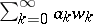, where the's are (usually) real numbers, and the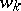's are the Walsh functions (either in Walsh's original order or in Paley's order). A Walsh–Fourier series is a Walsh series whose coefficients satisfy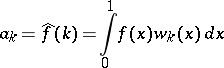for some integrable functionon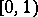. Key questions are: When does a Walsh–Fourier series approximate a function? When does a function have only one approximating Walsh series? And, how does the behaviour ofaffect the growth of the coefficients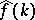?

The theory of Walsh series is distinguished from that of other orthonormal series (cf. also Orthonormal system) by three key features: 1) It has a close analogy with trigonometric series, owing to the fact that Walsh functions are piecewise-constant imitations of trigonometric functions, e.g.,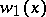imitatesand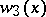imitates, and are the characters of the dyadic group, which can be identified with the interval, just like the complex trigonometric functions are the characters of the circle group, which can be identified with the interval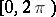. 2) Theth partial sum of any Walsh series forms a martingale. This opens the way for probabilistic techniques to be used on Walsh series, e.g., martingale convergence theorems and Hardy spaces without analytic functions. 3) It is the simplest of all orthogonal series, hence a good place to look for counterexamples. For example, P. Enflo used Walsh series to solve the basis problem by proving that there is a separable Banach space which has no basis.

Walsh series have many applications. For details and references see [a1], [a2] and [a3].

## Contents

### Approximation theory.

Since the Walsh–Fourier series of a functionusually converges toin some sense (e.g., uniformly, absolutely, in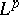norm, almost everywhere), Walsh series can be used to construct piecewise-constant approximations to functions.

### Image enhancement.

Since the number of jumps of a Walsh functionincreases asgets larger, higher-order Walsh–Fourier coefficients tend to be generated by sharp edges. Thus, one can reduce the contrast of an image by diminishing the higher-order terms of its Walsh–Fourier series, and increase contrast by diminishing the lower-order terms.

### Filtering.

By collecting data across several channels at once, one can obtain the Walsh–Fourier coefficients of a given signal while, at the same time, filter out most of its noise. This method is especially effective when the background noise is independent of the intensity of the signal, e.g., spectroscopy and radio astronomy.

### Data compression.

One can reduce the space needed to store or transmit a signal by using its Walsh–Fourier coefficients instead of the original signal, e.g., transmission of data through a photonic fibre optic cable.

### Optimization.

Genetic algorithms, methods for finding maxima and minima of non-differentiable functions, depend on Walsh functions for some of the theoretical development (cf. also Genetic algorithm).

### Modeling.

Walsh functions have been effective for modeling phenomena which have sharp transitions, such as shock waves from seismographs and visual patterns in a typical primate temporal cortex.